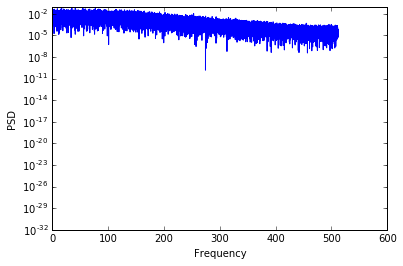# So much noise on a plane! Introducing the Power Spectral Density

##Power spectral density (PSD)

Wavy has been a little absent in the last period, engaged in several trips.
During last trip and reflecting about the sound he heard  on the plane and  the strange vibrations that resonate he wondered: how can we quantify and understand the noise that surrounds us?

One of the tool we have to characterize the ‘noise’ is through its Power Spectral Density. The Power spectral density function (PSD)  and therefore can show the strength of the variations (energy) as a function of frequency. Looking at the power spectral density of a time series we can understand where the contribution at some frequencies is higher and where lower,

So, PSD is a measure of a signal’s power intensity in the frequency domain and provides a useful way to characterize the amplitude versus frequency content of what we call ‘noise’.

### The mathematical representation

The one sided periodogram estimate  $$S_x(f)$$ of the  power spectral density (PSD) is defined in terms of the Fourier transform of a finite sample of data as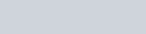$\displaystyle S_x[k] \equiv 2\times\frac{1}{T}\left|\hat{x}[k]\right|^2$

The division by $T$ is what turns an “energy” into a “power”; it also implies that the estimate at a given frequency will be independent on $T$.
The factor $2$ takes into account that we use only positive frequencies.

Note that the dimensions of the PSD: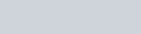$\displaystyle [S_x[k]]=[dt^2/T] \frac{1}{Hz}$

## Practical examples

Now, let’s use a bit of python code to show in practice how we can use the PSD of a signal.

In :
### importing the library
from __future__ import print_function
import numpy as np
import matplotlib.pyplot as plt
%matplotlib inline

from scipy.fftpack import fft


#### Let’s simulate a noise time series¶

In :
sampling=1024
nsample = 32768
dt = 1.0 / sampling
T = nsample * dt

x = np.linspace(0.0, nsample*dt,nsample)
nu=50.0# frequency in Hz of the sine function
sigma = 1.0
y = np.sin(nu * 2.0*np.pi*x) +sigma * np.random.normal(size=nsample)

fig, ax = plt.subplots()
ax.plot(x, y, label="Data")

Out:
[<matplotlib.lines.Line2D at 0x7f653b2b1f90>]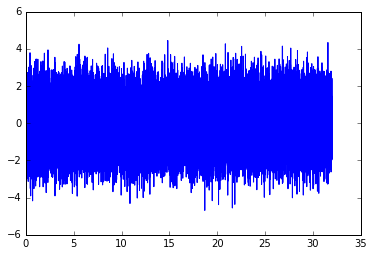#### …and perform its Fourier Transform¶

In :
yf = fft(y)
xf = np.linspace(0.0, 1.0/(2.0*dt), nsample/2)



The simple Power Spectral Density (One Sided, since we are considering only the positive Frequencies)
is

In :
# Let's check the (one sided) periodogram
oneSidedPeriodogram = 2/T*abs(yf[0:nsample/2])**2
plt.plot(xf,oneSidedPeriodogram)
plt.xlabel('Frequency')
plt.ylabel('PSD')

Out:
<matplotlib.text.Text at 0x7f6538c0d590>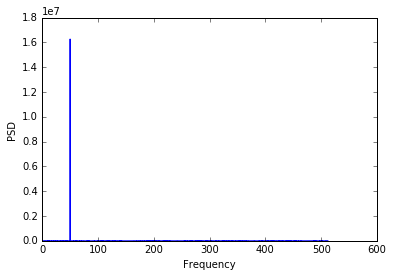### Using standard scipy library with its module signal¶

We obtain the same results, apart the normalization factor for the amplitude

In :

from scipy import signal

freqs, P_xx = signal.periodogram(y, sampling, scaling = 'density')
plt.plot(freqs, P_xx)
plt.xlabel('Frequency')
plt.ylabel('PSD')

Out:
<matplotlib.text.Text at 0x7f652ee72890>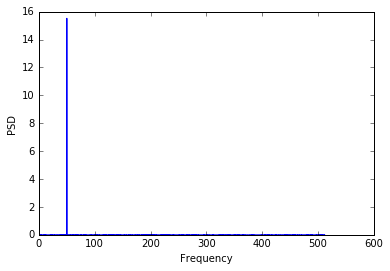So, in the plot we can clearly identify a Frequncy (the $50$ Hz) on a random noise. If in some way we can listen to these data, we will clearly hear a whistle at that frequency. What happen if we have a more featured surroinding noise?

Let’s simulate data using the model we introduced in our first post of these series, which was based on ARMA serie

In :
import statsmodels.api as sm
from statsmodels.tsa.arima_process import arma_generate_sample
np.random.seed(12345)

arparams = np.array([.75, -.25])
maparams = np.array([.65, .35])
arparams = np.r_[1, -arparams]
maparam = np.r_[1, maparams]
## simulate a simple sinusoidal function
x = np.linspace(0, 100, nsample)
y = arma_generate_sample(arparams, maparams, nsample)
fig, ax = plt.subplots()
ax.plot(x, y, label="Data")

Out:
[<matplotlib.lines.Line2D at 0x7f652c7a0f90>]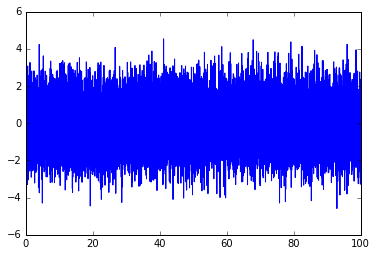### Now, how does look like its PSD?¶

In :

freqs, P_xx = signal.periodogram(y, sampling, scaling = 'density')
plt.plot(freqs, P_xx)
plt.xlabel('Frequency')
plt.ylabel('PSD')

Out:
<matplotlib.text.Text at 0x7f652b6f1c90>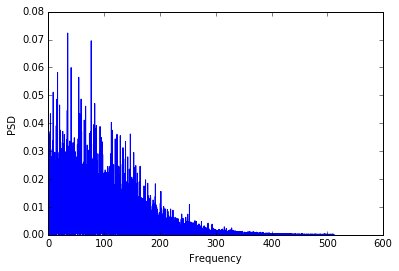We cannot recognize a single dominant contribution to a given frequency, but we found a forest of lines.
Each of these lines identify the intensity of the signal at the corresponding frequency

If we plot the data in a semilogaritmic plot, we can see better what we call a broad band noise. That is a noise where the spectrum of frequency contributing to it is very wide

In :
plt.semilogy(freqs, P_xx)
plt.xlabel('Frequency')
plt.ylabel('PSD')

Out:
<matplotlib.text.Text at 0x7f652edaee50>